# Using the Factor Theorem in Finding the Factors of Polynomials (With Examples)

• Author:
• Updated date:

Ray is a licensed engineer in the Philippines. He loves to write about mathematics and civil engineering.How to solve problems using the factor theoremPhoto by Jeswin Thomas on Unsplash

## The Factor Theorem

Factor theorem is a particular case of the remainder theorem that states that if f(x) = 0 in this case, then the binomial (x – c) is a factor of polynomial f(x). It is a theorem linking factors and zeros of a polynomial equation.

Factor theorem is a method that allows the factoring of polynomials of higher degrees. Consider a function f(x). If f(1) = 0, then (x-1) is a factor of f(x). If f(-3) = 0 then (x + 3) is a factor of f(x). The factor theorem can produce the factors of an expression in a trial and error manner. The factor theorem is useful for finding factors of polynomials.

There are two ways to interpret the factor theorem's definition, but both imply the same meaning.

### Definition 1

A polynomial f(x) has a factor x – c if and only if f(c) = 0.

### Definition 2

If (x – c) is a factor of P(x), then c is a root of the equation P(x) = 0, and conversely.

## Factor Theorem Proof

If (x – c) is a factor of P(x), then the remainder R obtained by dividing f(x) by (x – r) will be 0.

P(x) = (x – c) x Q(x)

Divide both sides by (x – c). Since the remainder is zero, then P(r) = 0.

P(x) / (x – c) = Q(x) + [0 / (x – c)

Conversely, if c is a root of P(x) = 0, P(c) = 0, then R = 0. Then,

P(x) / (x – c) = Q(x) + [0 / (x – c)]

P(x) = (x – c) x Q(x) + 0

P(x) = (x – c) x Q(x)

Scroll to Continue

Therefore, (x – c) is a factor of P(x).

## Example 1

### Factorizing a Polynomial by Applying the Factor Theorem

Factorize 2x3 + 5x2 – x – 6.

### Solution

Substitute any value to the given function. Say, substitute 1, -1, 2, -2, and -3/2.

f(1) = 2(1)3 + 5(1)2 - 1 – 6

f(1) = 0

f(-1) = 2(-1)3 + 5(-1)2 – (-1) - 6

f(-1) = -2

f(2) = 2(2)3 + 5(2)2 – (2) - 6

f(2) = 28

f(-2) = 2(-2)3 + 5(-2)2 – (-2) - 6

f(-2) = 0

f(-3/2) = 2(-3/2)3 + 5(-3/2)2 – (-3/2) - 6

f(-3/2) = 0

The function resulted to zero for values 1, -2, and -3/2. Hence using the factor theorem, (x – 1), (x + 2), and 2x +3 are factors of the given polynomial equation.

(x – 1), (x + 2), (2x + 3)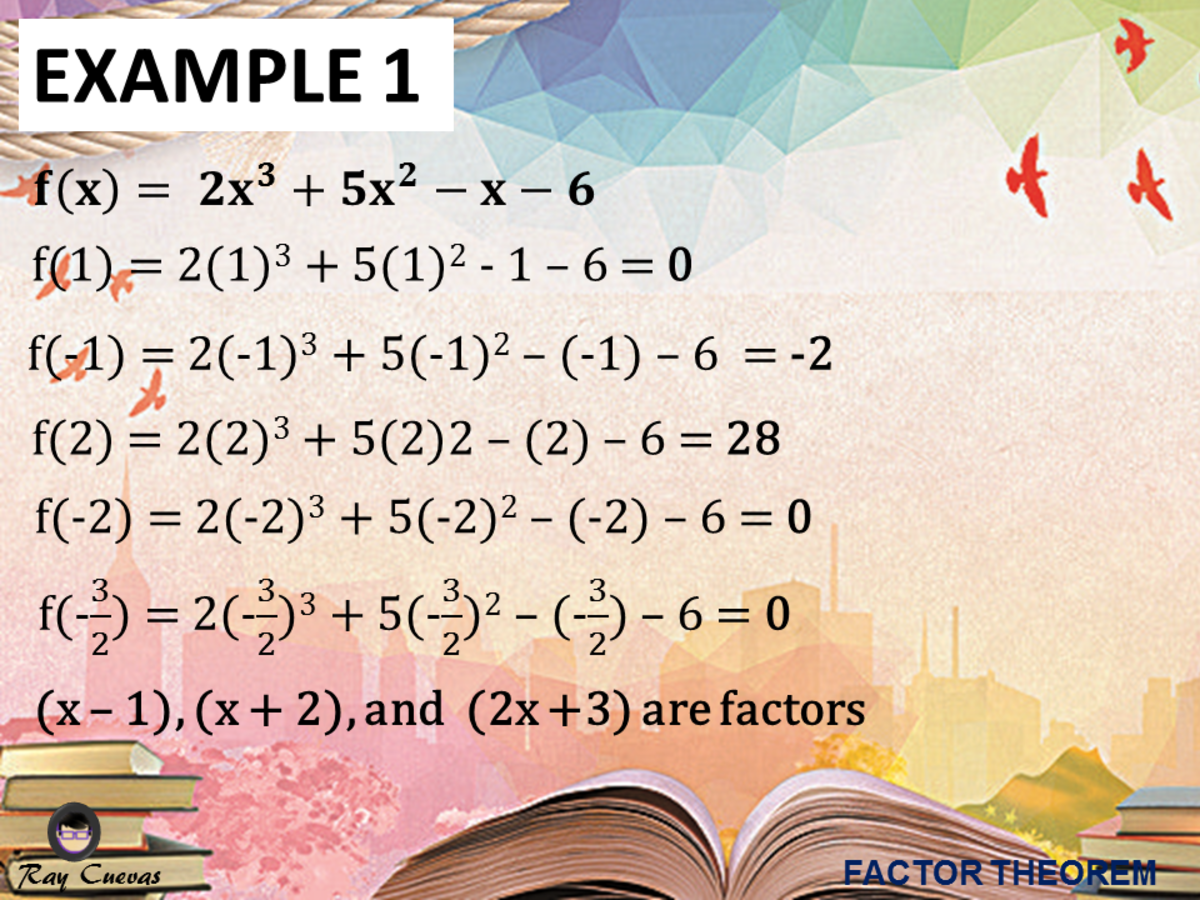Example 1: Factorizing a Polynomial by Applying the Factor TheoremJohn Ray Cuevas

## Example 2: Using the Factor Theorem

Using the factor theorem, show that x – 2 is a factor of f(x) = x3 – 4x2 + 3x + 2.

Solution

We need to show that x – 2 is a factor of the given cubic equation. Start by identifying the value of c. From the given problem, the variable c is equal to 2. Substitute the value of c to the given polynomial equation.

f(x) = x3 – 4x2 + 3x + 2

f(2) = (2)3 – 4(2)2 + 3(2) + 2

f(2) = 8 – 16 + 6 + 2

f(2) = 0

Since f(2) = 0, we see from the factor theorem that x – 2 is a factor of f(x). Another method would be to divide f(x) by x – 2 and show that the remainder is zero. The quotient in the division would be another factor of f(x).

## Example 3: Finding a Polynomial with Prescribed Zeros

Find a polynomial f(x) of degree 3 with zeros 2, -1, and 3.

Solution

By the factor theorem, f(x) has factors x – 2, x + 1, x -3.

f(x) = a (x – 2)(x + 1)(x – 3)

Thus, where any nonzero value may be assigned to a. If we let a = 1 and multiply to the factors, we obtain a polynomial of degree three. Same goes as you use different values of a since it will still be reduced to lowest and most simple equation.

f(x) = (x – 2)(x + 1)(x – 3)

f(x) = (x2 + x – 2x – 2)(x – 3)

f(x) = x3 + x2 – 2x2 – 2x – 3x2 – 3x + 6x + 6

f(x) = x3 – 4x2 + x + 6

The polynomial of degree 3 that has zeros 2, -1, and 3 is x3 – 4x2 + x + 6.

## Example 4: Proving an Equation Is a Factor of a Quadratic Equation

Show that (x + 2) is a factor of P(x) = x2 + 5x + 6 using the factor theorem.

Solution

Substitute the value of c = -2 to the given quadratic equation. Prove that x + 2 is a factor of x2 + 5x + 6 using the factor theorem.

P(-2) = (-2)2 + 5(-2) + 6

P(-2) = 4 – 10 + 6

P(-2) = 0

Therefore, x + 2 is a factor of x2 + 5x + 6.

## Example 5: Identifying if the Statement Is True Using Factor Theorem

Use the factor theorem to decide whether each statement is true.

a. x – 1 is a factor of x7 – 1.

b. x – 1 is a factor of 3x5 + 4x2 – 7.

c. x – 1 is a factor of x1992 – x1860 + x1754 – x1636.

d. x + 1 is a factor of x1992 – x1860 + x1754 – x1636.

Solution

a. To determine whether the statement is true, utilize the factor theorem and substitute c = 1 to the equation x7 – 1.

f(x) = x7 - 1

f(x) = (1)7 - 1

f(x) = 0

Since the result is zero, x – 1 is a factor of x7 – 1.

b. To determine whether the statement is true, utilize the factor theorem and substitute c = 1 to the equation x7 – 1.

f(x) = 3x5 + 4x2 – 7

f(x) = 3(1)5 + 4(1)2 – 7

f(x) = 3 + 4 - 7

f(x) = 0

Since the result is zero, x – 1 is a factor of 3x5 + 4x2 – 7.

c. To determine whether the statement is true, use the factor theorem and substitute c = 1 to the equation x1992 – x1860 + x1754 – x1636.

f(x) = x1992 – x1860 + x1754 – x1636

f(x) = (1)1992 – (1)1860 + (1)1754 – (1)1636

f(x) = 0

Since the result is zero, x – 1 is a factor of x1992 – x1860 + x1754 – x1636.

d. To determine whether the statement is true, use the factor theorem and substitute c = -1 to the equation

f(x) = x1992 – x1860 + x1754 – x1636

f(x) = (-1)1992 – (-1)1860 + (-1)1754 – (-1)1636

f(x) = 0

Since the result is zero, x + 1 is a factor of x1992 – x1860 + x1754 – x1636.

## Example 6: Proving X - C Is a Factor of a Function Given the Value of C

Use the factor theorem to show that x – c is a factor of f(x).

a. f(x) = x3 + x2 – 2x +12; c = -3

b. f(x) = x12 – 4096; c = -2

c. f(x) = x4 – 2x3 + 3x – 36; c = 3

d. f(x) = x3 + x2 – 11x + 10; c = 2

e. f(x) = x5 + 1024; c = -4

Solution:

a. Using the factor theorem, substitute the value of c = -3 to the given cubic function f(x) = x3 + x2 – 2x +12.

f(x) = (-3)3 + (-3)2 – 2(-3) +12

f(x) = -27 + 9 + 6 + 12

f(x) = 0

Therefore, x + 3 is a factor of x3 + x2 – 2x +12.

b. To show that x + 2 is a factor of f(x) = x12 – 4096, substitute the value of c to the given polynomial equation.

f(x) = x12 – 4096

f(x) = (-2)12 – 4096

f(x) = 0

Therefore, x + 2 is a factor of the polynomial equation x12 – 4096.

c. Using the factor theorem, substitute the value of c = 3 to the given cubic function f(x) = x4 – 2x3 + 3x – 36.

f(x) = x4 – 2x3 + 3x – 36

f(3) = (3)4 – 2(3)3 + 3(3) – 36

f(3) = 0

Since the resulting answer is zero, x – 3 is a factor of x4 – 2x3 + 3x – 36.

d. Using the factor theorem, substitute the value of c = 2 to the given cubic function f(x) = x3 + x2 – 11x + 10.

f(x) = x3 + x2 – 11x + 10

f(2) = (2)3 + (2)2 – 11(2) + 10

f(2) = 0

Since the resulting answer is zero, x – 2 is a factor of x3 + x2 – 11x + 10.

e. Using the factor theorem, substitute the value of c = -4 to the given cubic function f(x) = x5 + 1024.

f(x) = x5 + 1024

f(-4) = (-4)5 + 1024

f(-4) = 0

Since the resulting answer is zero, x + 4 is a factor of x5 + 1024.

## Example 7: Finding the Equation Given the Zeros with the Use of Factor Theorem

Use the factor theorem to find the polynomial equation of degree 4 given the zeros -2, -1, 1, and 4.

Solution

Given the zeros -2, -1, 1, and 4, you can use the factor theorem’s definition to get the factors. Also, given the degree of 4, there should be 4 factors.

(x + 2)(x + 1)(x – 1)(x – 4)

Use the FOIL Method and multiply (x + 1) and (x – 1) first.

(x + 1)(x – 1)

(x + 2)(x2 – 1)(x – 4)

Next, utilize the FOIL Method and multiply (x + 2) and (x – 4).

(x + 2) (x – 4)

(x2 – 1)(x2 – 2x – 8)

Simplify the equation by using the distributive property of multiplication.

x4 – 2x3 – 8x2 – x2 + 2x + 8

x4 – 2x3 – 9x2 + 2x + 8

The resulting polynomial equation in the 4th degree is x4 – 2x3 – 9x2 + 2x + 8.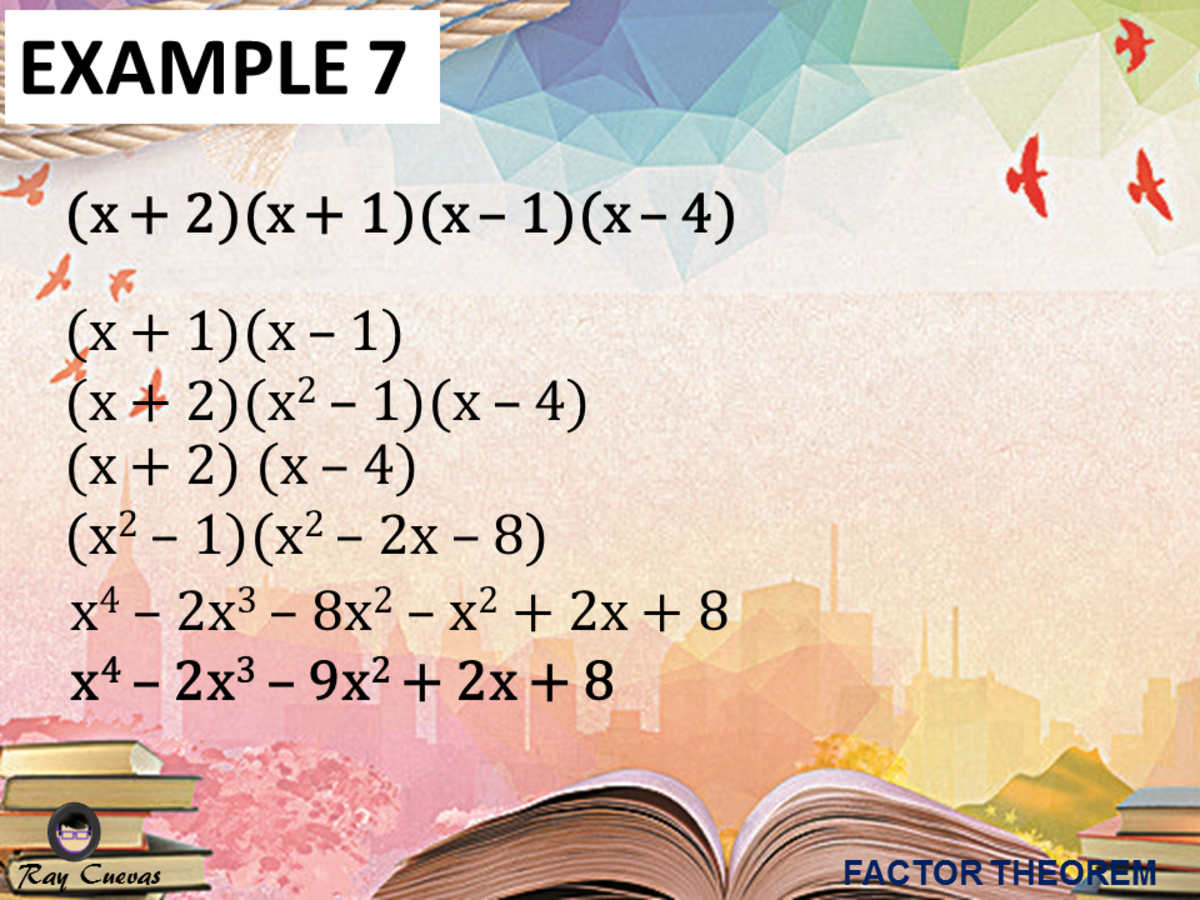Example 7: Finding the Equation Given the Zeros with the Use of Factor TheoremJohn Ray Cuevas

## Example 8: Determining if X - C Is a Factor of an Equation

Determine if x + 3 is a factor of the equation f(x) = x3 + x2 – 2x + 12.

Solution

Use the factor theorem and plug in the given value of c = -3.

f(x) = x3 + x2 – 2x + 12

f(-3) = (-3)3 + (-3)2 – 2(-3) + 12

f(-3) = 0

Therefore, f(-3) = 0 so x + 3 is a factor of f(x) = x3 + x2 – 2x + 12.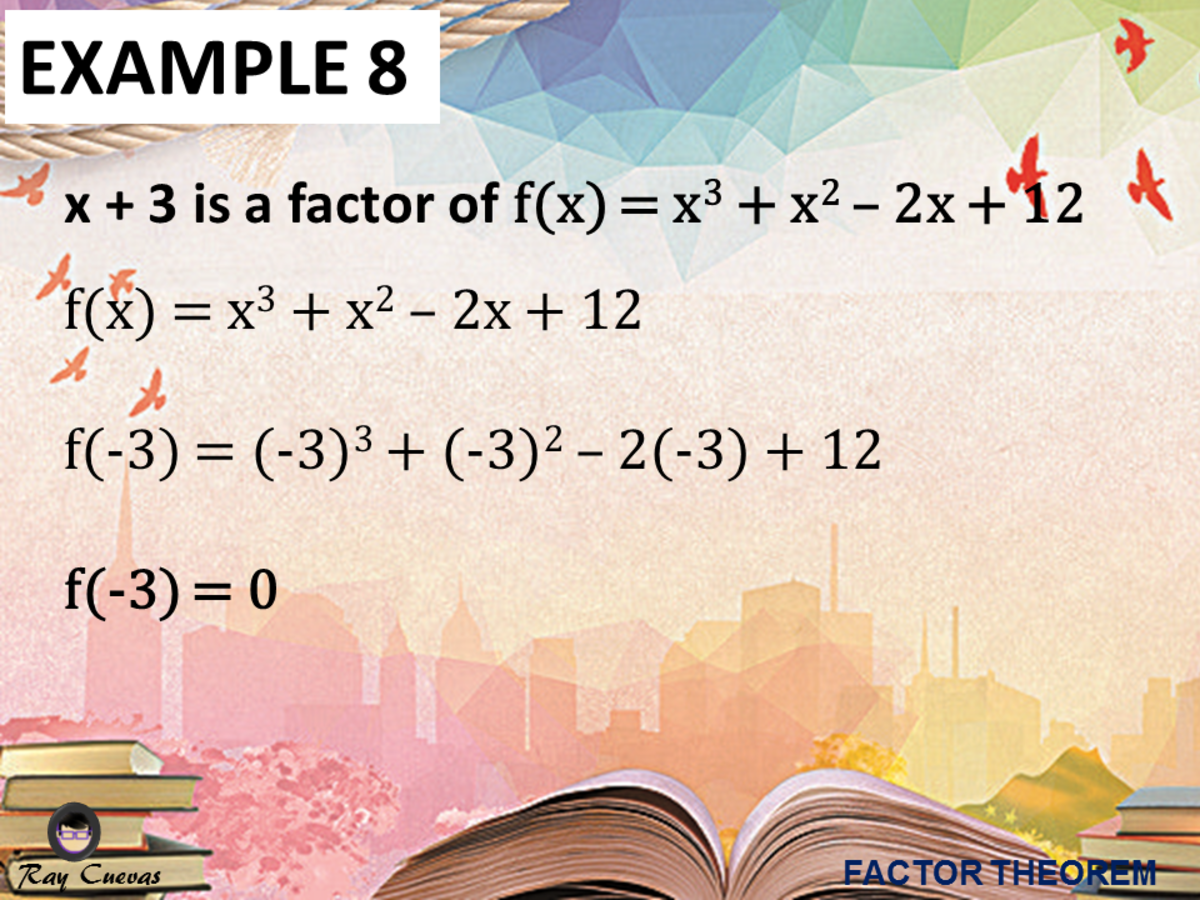Example 8: Determining if X - C Is a Factor of an EquationJohn Ray Cuevas

## Example 9: Determining if X – C Is a Factor of an Equation

Determine if x - 2 is a factor of the equation f(x) = x4 – 3x3 – 2x2 + 5x + 6.

Solution

Use the factor theorem and plug in the given value of c = 2.

f(x) = x4 – 3x3 – 2x2 + 5x + 6

f(2) = (2)4 – 3(2)3 – 2(2)2 + 5(2) + 6

f(2) = 16 – 3(8) – 2(4) + 10 + 6

f(2) = 16 – 24 – 8 + 10 + 6

f(2) = 0

Therefore, f(2) = 0 so x - 2 is a factor of f(x) = x4 – 3x3 – 2x2 + 5x + 6.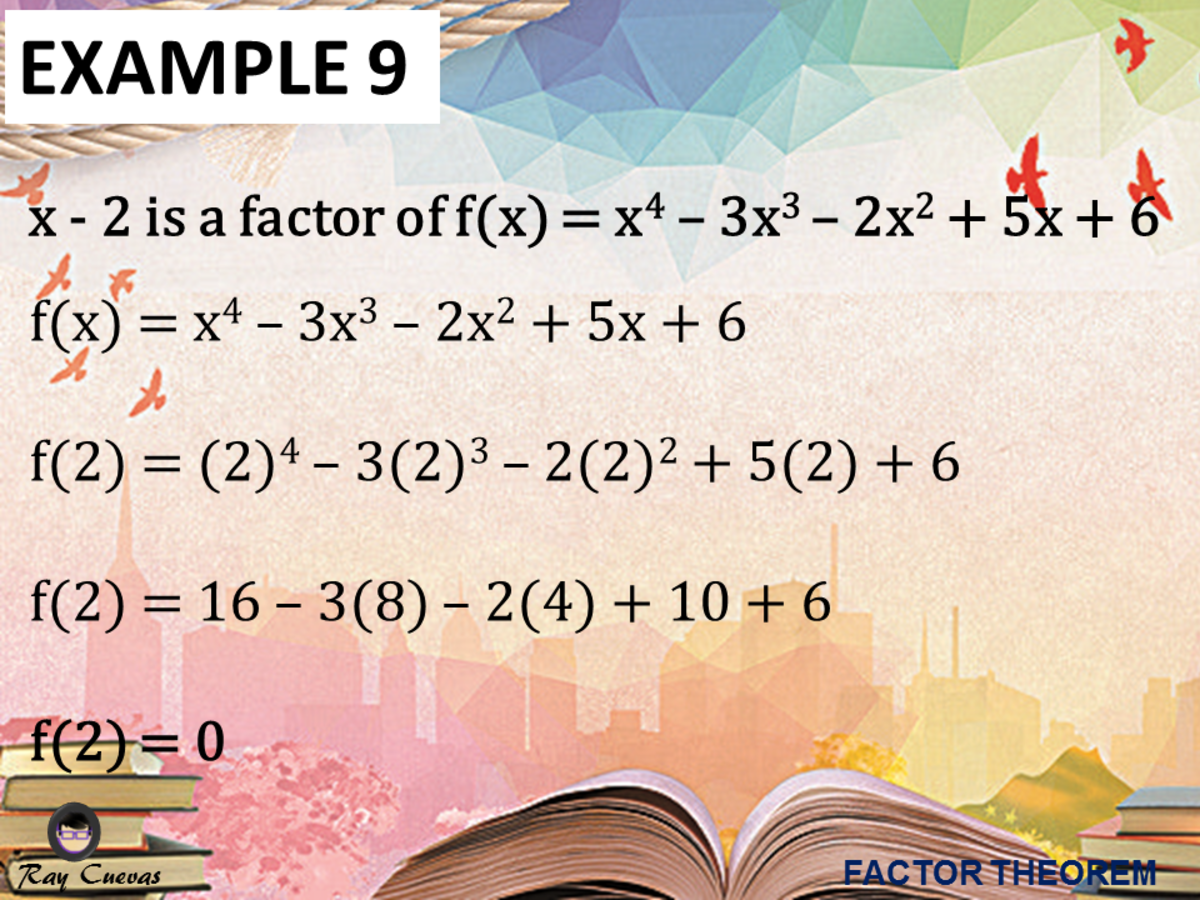Example 9: Determining if X – C Is a Factor of an EquationJohn Ray Cuevas

## Example 10: Finding the Polynomial Equation Given the Zeros

Use the factor theorem to find the polynomial equation of degree 3 given the zeros -2, 0, and 5.

Solution

Given the zeros -2, 0, and 5, you can use the factor theorem’s definition to get the factors. Also, given the degree of 3, there should be 3 factors.

(x + 2)(x)(x – 5)

Distribute x to x – 5.

(x + 2)(x2 – 5x)

Next, utilize the FOIL Method and foil the polynomials (x + 2) and (x2 – 5x).

(x + 2) (x2 – 5x)

(x3 – 5x2 + 2x2 – 10x)

Simplify the equation by adding or subtracting like terms.

x3 – 5x2 + 2x2 – 10x

x3 – 3x2 – 10x

The resulting polynomial equation in the 3rd degree is x3 – 3x2 – 10x.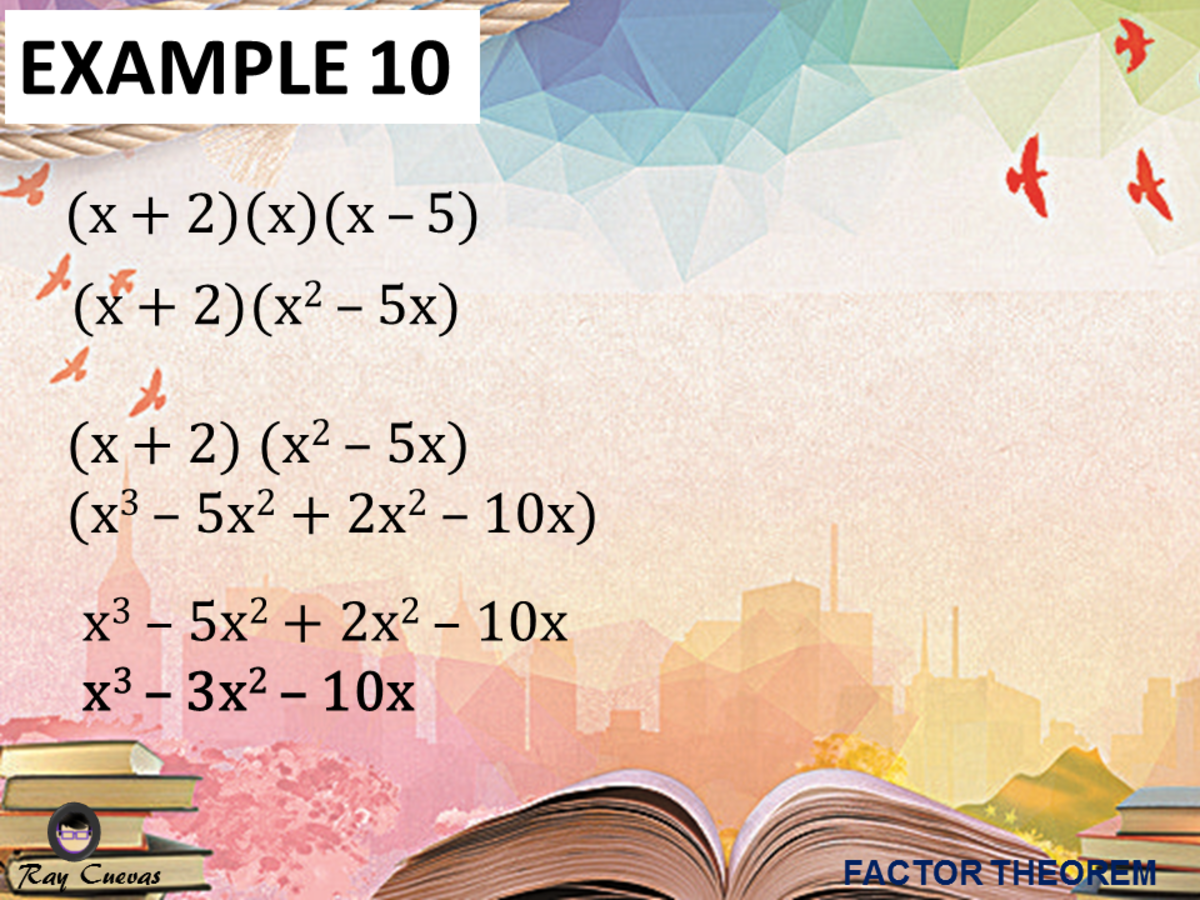Example 10: Finding the Polynomial Equation Given the Zeros John Ray Cuevas

## Explore Other Math Articles

This content is accurate and true to the best of the author’s knowledge and is not meant to substitute for formal and individualized advice from a qualified professional.